# Coulomb law

It states that the force of attraction or repulsion between two stationary point charges is

1. Directly proportional to the product of the magnitudes of the two charges and
2. Inversely proportional to the square of the distance between them

This force acts along the line joining the two charges.

If two point charges q1 and q2 are separated by distance r, then the force F of attraction or repulsion between them is such that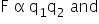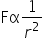where k is constant of proportionality called electrostatic force constant.

In free space, we have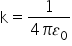= 9*109 Nm 2C-2

Where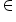0 is called permittivity of the free space. So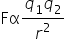Units of charge:

1. The SI unit of charge is coulomb.

If q1 and q2 = 1C and  r = 1m, then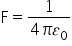= 9*109 N

So one coulomb is that amount of charge that repels an equal and similar charge with a force of 9*109 N when placed in vacuum at a distance of 1m from it.

1. In electrostatic CGS system, the unit of charge is known as electrostatic unit of charge or statcoulomb.

1 coulomb = 3 *109 statcoulomb

1. In electromagnetic CGS system, the unit of charge is abcoulomb  or emu.

1 coulomb = 1/ 10 abcoulomb

Coulomb law in vector form:

As shown in fig. consider two positive point charges q1 & q2 placed in vacuum at distance r from each other. They repel each other.

In vector form, coulomb’s law may be expressed as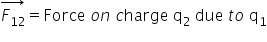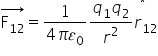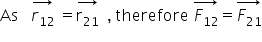Permittivity : it is the property of the medium which determines the electric force between two charges situated in that medium.

Dielectric constant or relative permittivity :

According to coulomb’s law , the force between two point charges q1 & q2, placed in vacuum at distance r from each other, is given by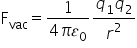…………… (1)

When the same two charges are placed same distance apart in any medium other than vacuum, the force them becomes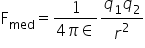Dividing 1 by 2, we getThe ratio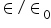of the permittivity (ε0) of the medium to the permittivity (ε0) of free space is called relative permittivity (ε0) or dielectric constant K of the given medium. Thus,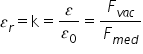k(vacuum) = 1

k(air) = 1.00054

k(water) = 80

Principle of superposition of electrostatic forces:

This state that when a number of charges interacting, the total force on a given charge is the sum of the forces exerted on it due to all other charges. The force between two charges is not affected by te presence of other charges.

As shown in fig. consider N point charge q1, q2, q3………….qn, placed in vacuum at points whose position vectors w.r.t. origin o are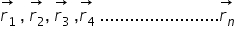respectively. According to the principle of superposition, the total force on charge Q is given by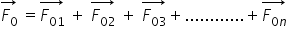Where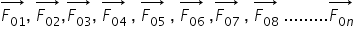are the forces exerted in

charge Q by the individual charges Q1, Q2, Q3 …………Qn respectively.

According to coulomb’s law, the force exerted on charge q1 due to q2 is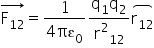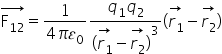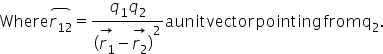Hence the total force on charge q1 is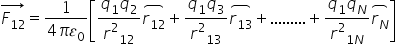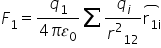In terms of position vectors,

Related Keywords